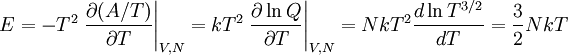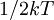Ideal gas: Energy$E = -T^2 \left. \frac{\partial (A/T)}{\partial T} \right\vert_{V,N} = kT^2 \left. \frac{\partial \ln Q}{\partial T} \right\vert_{V,N}= NkT^2 \frac{d \ln T^{3/2}}{dT} = \frac{3}{2} NkT$
This energy is all kinetic energy,$1/2 kT$ per degree of freedom, by equipartition. This is because there are no intermolecular forces, thus no potential energy.